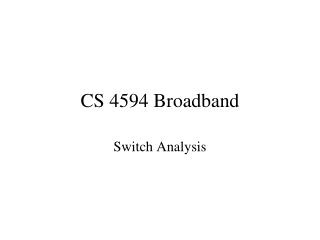# CS 4594 Broadband - PowerPoint PPT PresentationDownload PresentationDownload Presentation- - - - - - - - - - - - - - - - - - - - - - - - - - - E N D - - - - - - - - - - - - - - - - - - - - - - - - - - -
##### Presentation Transcript

1. CS 4594 Broadband Switch Analysis

2. Diagram of Buffered Switch N outputs N inputs buffer buffer buffer buffer buffer buffer buffer buffer buffer

3. General Queuing Assumptions For this NxN switch: Assume that at each clock cycle, cells arrive evenly on each input, say with probability p. Assume the probability of each incoming cell on any input going to any particular output is the same. Then the probability of a cell arriving at input i and going to output j is p/N. What is the probability that m cells arrive at output j at the same time?

4. Diagram of Input Buffered Switch N outputs N inputs buffer buffer buffer

5. Reference • Chao, Lam, Oki, Broadband Packet Switching Technologies, Wiley, 2001. • De Prycker, Asynchronous Transfer Mode, 3rd Edition, Prentice Hall, 1995.

6. Assumptions for Input-Buffered • N inputs and N outputs • Queues are FIFOs • Of all the cells destined for a particular output, only one cell can pass through the switch at a time • As soon as a cell is taken from the head of the line (HOL) a cell replaces it (saturation)

7. Assumptions for Input Buffered Switch • N = number of inputs. • Ai[m] = number of cells arriving at the heads of the queues during the mth time slot and destined for output i. • Bi[m]= number of remaining cells at input buffers destined for output i in the mth time slot. • Bi[m]= max (0, Bi[m-1]+ Ai[m] –1) • F[m] = number of cells transported through switch during mth time slot

8. Equations for Input Queue

9. Results for Input Queue Poisson Rate: Steady state:

10. Results for Input Queue

11. Input Queuing For saturated switch with an infinite number of inputs Pmax = 0.586

12. Diagram of Output Buffered Switch N outputs N inputs buffer buffer buffer

13. Queuing Assumptions The probability of a cell arriving at input i and going to output j is p/N. What is the probability that m cells arrive at output j at the same time?

14. The Answer Probability i cells arrive at a certain output queue = Comb(i, N) (p/N)i (1- p/N)N-i Here Comb(i, N) is the combination number of how many ways a group of i items can be chosen from a set of N elements.

15. Markov Chains • Markov Chain: Description of a system in terms of a set of states. The system satisfies the Markov assumption: The future state of the system depends only upon its current state. • Each state is assigned a probability and transition from one state to another is determined by a fixed set of transition probabilities.

16. Assumptions for Output Buffered • Ak = Prob[A = k] = Comb(k,N) (p/N)k (1-p/N)N-k • Let Q[m] be the number of cells in the ith queue at the end of the mth time slot • Q[m] = min(max(0, Q[m-1] + Am – 1), b) A4 A4 A3 A3 A3 A2 A2 A2 A2 A3 A1 A1 A1 A1 0 1 2 3 A0 A0 A0 A0

17. Markov Chain for Output Buffered • Ak = Prob[A = k] = Comb(k,N) (p/N)k (1-p/N)N-k • P[i,j]=Pr[Q[m]=j | Q[m-1]=i] A4 A4 A4 A3 A3 A3 A2 A2 A2 A2 A3 A1 A1 A1 A1 0 1 2 3 A0 A0 A0 A0

18. Assumptions for Output Buffered • P[i,j]=Pr[Q[m]=j | Q[m-1]=i] P[0,0]=A0+A1 P[0,1]=A2 P[0,2]=A3 … P[0,N]=AN+AN+1+AN+2+…+AM P[1,0]=A0 P[1,1]=A1 P[1,2]=A2 … P[1,N]=AN-1+AN+AN+1+…+AM P[2,0]=0 P[2,1]=A0 P[2,2]=A1 … P[2,N]=AN-2+AN-1+AN+…+AM … … P[N,0]=0 … P[N,N-1]=A0 P[N,N]=A1+A2+…+AM A4 A4 A4 A4 A3 A3 A3 A3 A2 A2 A2 A2 A1 A1 A1 A1 0 2 3 1 A0 A0 A0 A0

19. In and Out

20. Solving

21. Generating Function • Let • xi = Comb(i, N) (p/N)i (1- p/N)N-i • Then • xi zi , for i = 0, 1, 2, . . ., N = (z (p/N) + (1-p/N))N

22. Average Queue Size It can be shown that the average queue size is: Q = (N-1)/N p2 /(2 (1-p)) And the average waiting time is W = (N-1)/N p /(2 (1-p))

23. Graph of Results for N=2, 4, 8, 16 4.5 4 3.5 3 2.5 2 1.5 1 0.5 0 0.1 0.2 0.3 0.4 0.5 0.6 0.7 0.8 0.9

24. Central Queuing • Central Queues behave like output queues according to de Precker